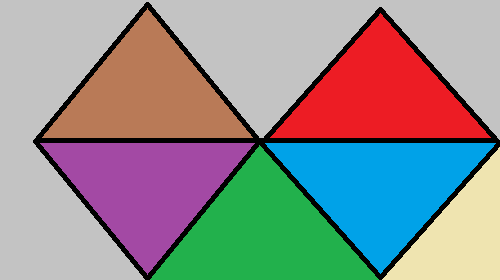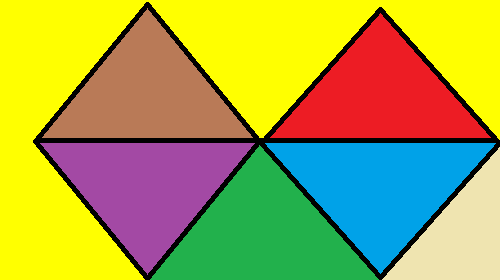# Floodfill Image using Python-Pillow

Seed Fill also known as flood fill, is an algorithm used to identify connected paths in a definite enclosed region. The algorithm has an array of practical applications, such as –

• Optimized pathfinding
• Paint Bucket Tool a generic tool found in several image processing packages, uses the algorithm internally
• Mazesolving uses floodfill (paired with traversing algorithms like breadth-first, depth-first and pathfinding algorithms such as A Star, Dijkstra)
• Used in Image Processing

There are various ways in which the algorithm could be implemented such as –

• Scanline Floodfill (row/column based floodfill)
• Four/Eight Way Floodfill
• Threshold less Floodfill (using only identical pixel values)

We will be utilizing floodfill algorithm in order to do image processing tasks. For this purpose, we will be using `pillow` library. To install the library, execute the following command in the command-line:-

```pip install pillow
```

Note: Several Linux distributions tend to have Python and Pillow preinstalled into them

Syntax: ImageDraw.floodfill(image, seed_pos, replace_val, border-None, thresh=0)

Parameters:
image – Open Image Object (obtained via Image.open, Image.fromarray etc).
seed_pos – Seed position (coordinates of the pixel from where the seed value would be obtained).
replace_val – Fill color (the color value which would be used for replacement).
border – Optional border value (modifies path selection according to border color)
thresh – Optional Threshold Value (used to provide tolerance in floodfill, to incorporate similar valued pixel regions)

Return: NoneType (modifies the image in place, rather then returning then modified image)

Example:

Image Used:`# Importing the pillow library's  ` `# desired modules ` `from` `PIL ``import` `Image, ImageDraw ` `  `  `# Opening the image (R prefixed to ` `# string in order to deal with '\' ` `# in paths) ` `img ``=` `Image.``open``(R``"sample.png"``) ` ` `  `# Converting the image to RGB mode ` `img1 ``=` `img.convert(``"RGB"``)  ` ` `  `# Coordinates of the pixel whose value ` `# would be used as seed ` `seed ``=` `(``263``, ``70``) ` `  `  `# Pixel Value which would be used for ` `# replacement  ` `rep_value ``=` `(``255``, ``255``, ``0``) ` `  `  `# Calling the floodfill() function and  ` `# passing it image, seed, value and  ` `# thresh as arguments ` `ImageDraw.floodfill(img, seed, rep_value, thresh``=``50``) ` `  `  `# Displaying the image ` `img.show() `

Output:Explanation:

• After importing the necessary modules required for the task, we firstly create an image object (`'PIL.Image.Image'`). This image objects acts as an separate in-core copy of the Image file, which could be used separately.
• Then assign a coordinate value (inside dimensions of the image) for the seed variable. The Coordinates are picked manually, i.e. the user should put in the value of coordinate which is picked intentionally (the value of the pixel coordinate could be verified by using `img.getpixel(coord)`).
• The pixel value obtained from these coordinates would be the one which is to be replaced inside the image.
• Then assign `rep_value` variable with a RGB color value (yellow in this case). The value is being assigned as a RGB Tuple, which is specific for our particular case as our input image is of RGB color space (`img.mode == 'RGB'`).

Note: The rep_value variable will contain value according to the Image mode of the current image, i.e. if `img.mode == "L"` then rep value will not be of tuple with 3 components, but rather would be of integer.

• Then call the `ImageDraw.floodfill()` function by passing img, seed, rep_value and thresh as arguments. Since the `ImageDraw.floodfill() ` function modifies the passed image object at place, we don’t need to store the return value (Nonetype) of the function.
• In the end we display the modified image, using `img.show() `(Image.show()).

My Personal Notes arrow_drop_upCheck out this Author's contributed articles.

If you like GeeksforGeeks and would like to contribute, you can also write an article using contribute.geeksforgeeks.org or mail your article to contribute@geeksforgeeks.org. See your article appearing on the GeeksforGeeks main page and help other Geeks.

Please Improve this article if you find anything incorrect by clicking on the "Improve Article" button below.

Article Tags :

Be the First to upvote.

Please write to us at contribute@geeksforgeeks.org to report any issue with the above content.